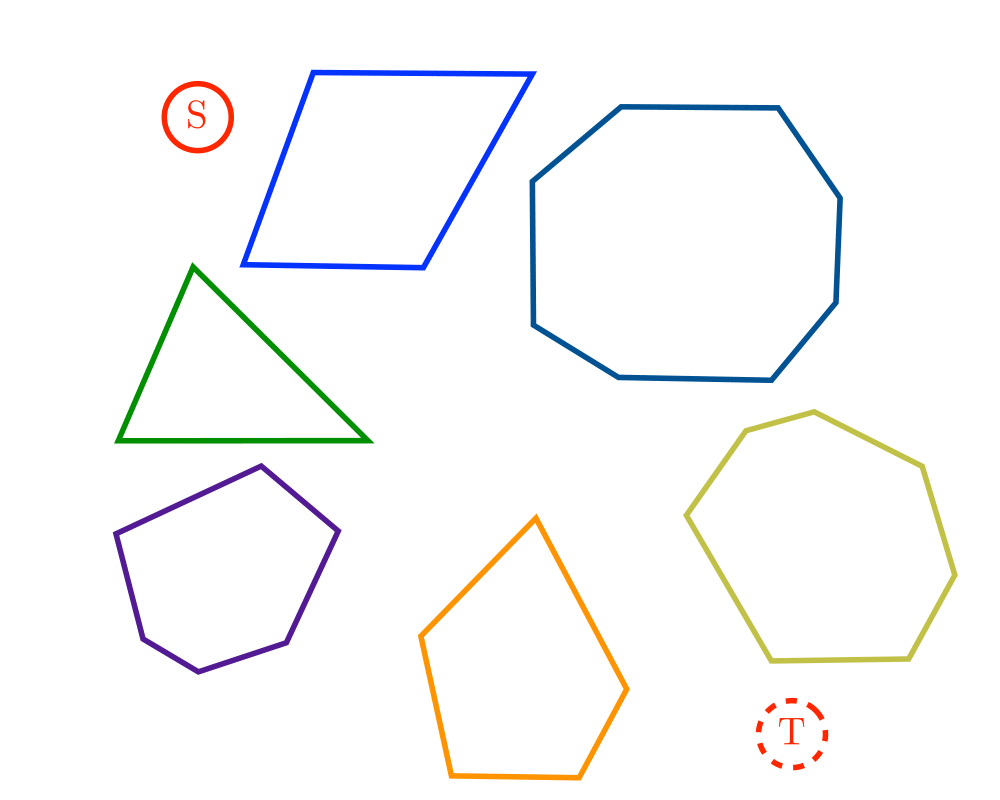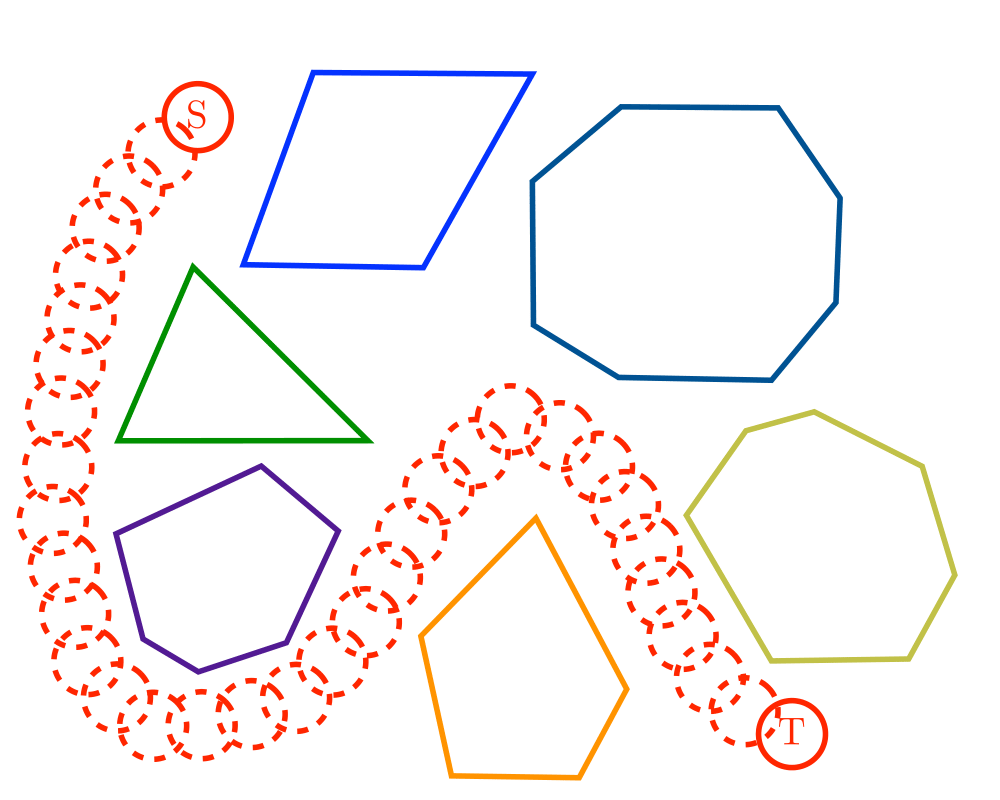# Motion PlanningInputOutput

Input Description: A polygonal-shaped robot $$s$$ in a given starting position in a room containing polygonal obstacles, with a desired ending position $$t$$.
Problem: Find the shortest path in the room taking $$s$$ to $$t$$ without going through any of the obstacles.

Excerpt from The Algorithm Design Manual: The difficulty of motion planning will be obvious to anyone who has ever had to move a large piece of furniture into a small apartment. The problem of motion planning also arises in systems for molecular docking. Many drugs are small molecules that act by binding to a given target model. The problem of identifying which binding sites are accessible to a candidate drug is clearly an instance of motion planning. Plotting paths for mobile robots is another canonical motion-planning application.

Motion planning also provides a tool for computer animation. Given a set of object models that appear in two different scenes $$s1$$ and $$s2$$, a motion planning algorithm can construct a short sequence of intermediate motions to transform $$s1$$ to $$s2$$. These motions can serve to fill in the intermediate scenes between $$s1$$ and $$s2$$, with such scene interpolation greatly reducing the amount of work the animator has to do.

Go To Main Page